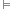# Conditional

The conditional, material conditional or material implication operator is used to symbolize “if… then” statements in symbolic logic. The conditional is a truth-functional operator in logic.

The symbol is used to indicate a conditional relationship, though some systems use instead.

## Example

“If P, then Q” may be formalized as P → Q.

## Truth Values

The following truth table illustrates the possible truth values for a conditional expression P → Q, given all possible valuations for propositions P and Q. Specifically, P → Q is only false on the valuation that P is true and Q is false.

P Q P → Q
T T T
T F F
F T T
F F T

## Differences between → and the English if… then

It is noteworthy that a conditional operation is always true if its consequent (the part following the operator) is true. This is not normally considered to be the case in English “if…then” statements, because the → operator is not really an if…then operator.

For example, let P be “The Earth orbits the moon” and let Q be “the Earth orbits the Sun”.

In symbolic logic, the statement P → Q in this case is true. However, it translates into English as “If the Earth orbits the moon, then the Earth orbits the Sun”. This intuitively seems to be a false statement, because the antecedent (P) is false (the Earth does not orbit the moon). In logic, however, this operator always produces a true formula if the consequent (Q) is true, even if the antecedent is false.

Similarly, if P instead was “All canines are mammals”, we come to an odd statement. P → Q would still be true, but the English version is now “If all canines are mammals, then the Earth orbits the Sun”. Even though both the antecedent (P) and conseqent (Q) are true in this version, we may be hesitant to claim that the resulting English expression is true, since we expect there to be some dependency expressed by an if…then statement, otherwise it would be meaningless to even state the antecedent if we mean only to say that the Earth orbits the Sun.

Finally, if P and Q are both false, P → Q is true. This again causes confusion in English, since the phrase “If the Earth is flat, then penguins speak English” would be counted as true if symbolized.

Because of these problems, we may more accurately translate the conditional expression P → Q into English by saying “it is not the case that P is true and Q is false“. This may be translated back into symbolic logic as ¬(P ∧ ¬Q) or ¬P ∨ Q, both of which turn out to be logically equivalent to P → Q.

## Material and Logical Implication

The function of the conditional operator is also known as material implication. This should not be confused with logical implication ().

The relationship of material implication, P → Q, states that as long as P is true, then Q is true, or in other words, it is never true that P is true and Q is false. In contrast, the logical implication PQ states that P is the case, and therefore, Q is the case. The logical implication PQ states that the material implication formula P → Q is always true, but the logical implication () has no truth value itself, because it describes the relation between formulae, or sets of formulae, rather than any truth-functional operation of them.

In order to avoid confusion, P → Q is usually read as “If P, then Q” rather than “P implies Q” or “P, therefore Q”, those being reserved for logical implication.